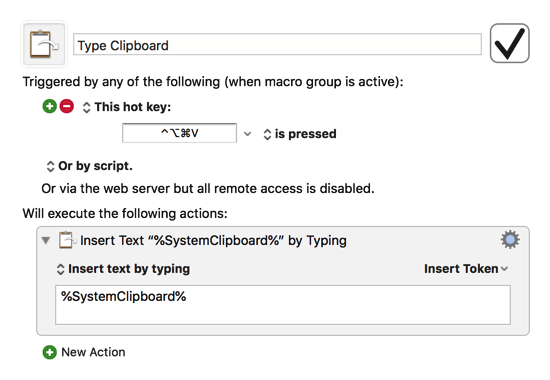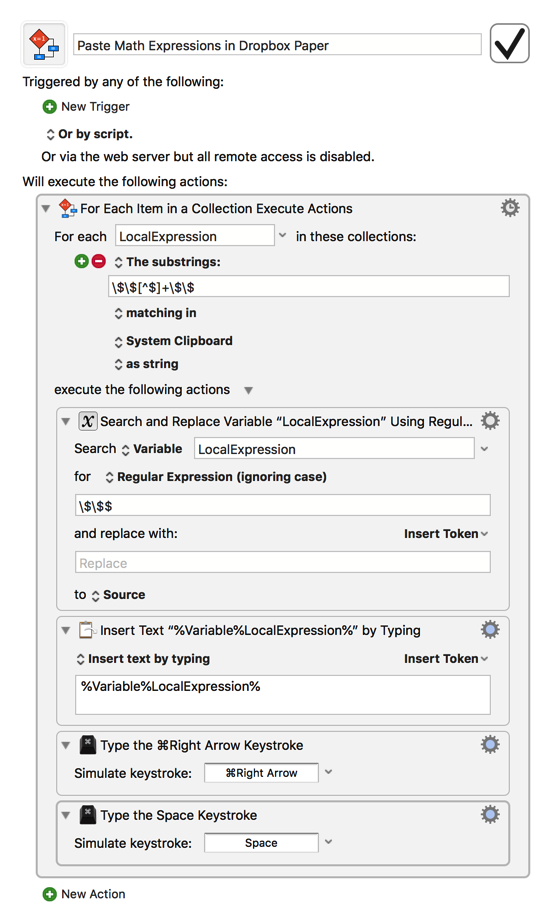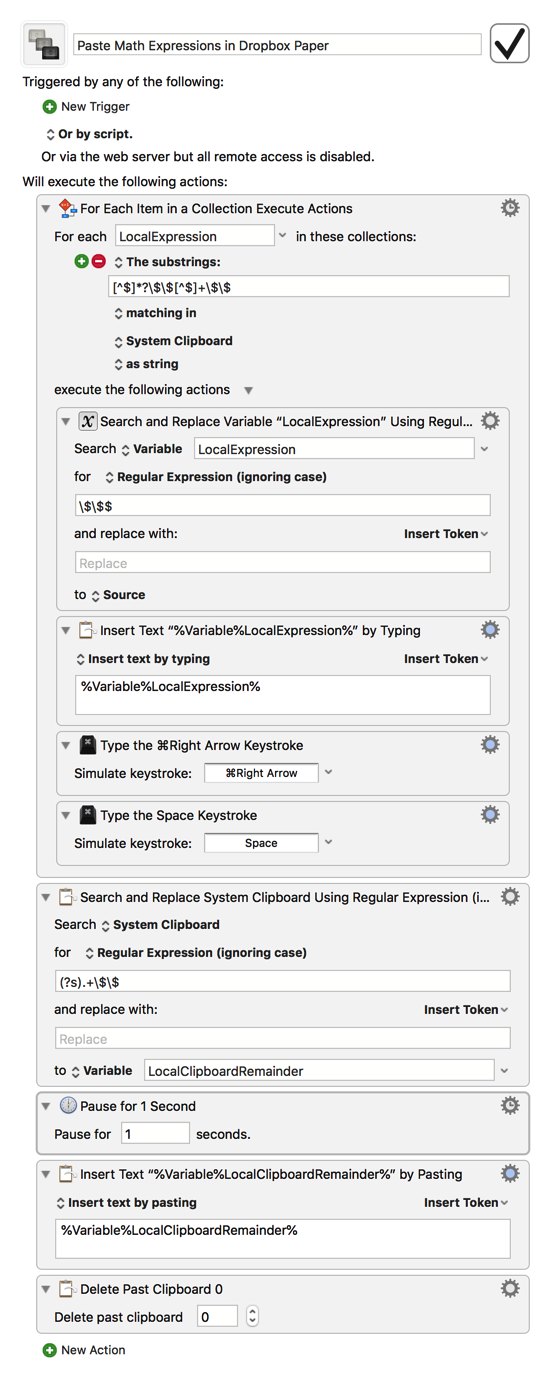# Improving Dropbox Paper LaTeX support with Keyboard Maestro

Dropbox Paper has basic LaTeX support. If you type $$math$$ then the math is rendered using KaTeX.

However copying and pasting LaTeX into Dropbox Paper does not work so well, as in order for Dropbox Paper to recognise that you are typing maths, you have to first type $$and then wait a moment before typing the maths. This is easiest to explain with a .gif:As the gif shows, the copy-pasted$$ a^2 + b^2 = c^2$$does not render. Is it possible to create a macro that will take a piece of copied text, and go through it sequentially, searching for a$$ math $$expression, removing it, typing$$, waiting a moment, then pasting the ‘math’, and so on?

Before exploring that solution, I'm curious: have you tried creating a simple macro that inserts the current clipboard contents via typing instead of via pasting, like this?Or does the LaTeX only render when there's a pause after typing $$? EDIT: I just tried this out myself, and inserting the clipboard via typing does seem to work as long as you only include the first$$. However, since the parsing doesn't seem to take place until after the cursor exits the pair of $$, you may also want to add a ⌘→ keystroke followed by a space after inserting the clipboard by typing to help further automate the parsing. 1 Like Thanks! Pasting as typing correctly makes Dropbox realise LaTeX is being entered, but due to the way Dropbox interprets math environments it doesn’t work properly. Dropbox expects: • User types $$
• Dropbox opens math environment, adds a closing $$ and places the cursor inside the pair $$ ... $$ • User types some maths, eg a^2 + b^2 = c^2 and then uses the right arrow key to move outside the closing $$.

So if my clipboard has $$a^2 + b^2 = c^2$$ on it then pasting that while typing results in an additional $$ at the end, and leaves the cursor inside the math environment. That I suppose is easily fixed: I can take the clipboard, remove the last $$, paste the clipboard while typing and then advance the cursor one space forwards.

This would solve the issue for adding an individual math expression—thank you again!

However ideally what I’d like is to be able to paste a (potentially large) text, and have it run through the text and do this for each separate math expression (of which there could be many). Is this possible with KM?

EDIT: sorry didn’t see you’d edited your comment!

Certainly, but we need to be able to tell KM how to find each separate math expression. How would multiple expressions be separated in a single large text? Would each one just be surrounded by $$on each side, in which case the solution should be fairly easy, or is it something else? 1 Like Yes, each individual expression would be of the form$$ math $$. Okay then, here you go: Paste Math Expressions in Dropbox Paper.kmmacros (3.2 KB)You could optionally add an If/Then check at the top to make sure it only works in Paper, but for now, I've given this a couple of quick tests and it seems like it should do the trick. 1 Like Thank you very much! That doesn’t quite do what I want it to, but that’s my fault for being unclear. When I run that on a file with multiple math expressions, it strips out everything but the math, i.e. it converts:$$math1$$text$$math2$$text$$math3$$into$$math1math2math3

whereas what I want is for it not to remove the ‘text’ in between the math expressions (so that I can take a LaTeX document written elsewhere and paste it into Dropbox Paper). Is that harder to achieve?

Yes, but fortunately not much so. First we need to change the regex used to find expressions to include all the text that comes before each expression, so that nothing gets left out when each one is pasted, then we need to erase all the text leading up to and including the final expression from the clipboard so that we can paste the remainder of the text without including any expression twice. In my tests, this also requires a pause of about 1 second to prevent the original clipboard contents from being pasted; fortunately, in practice it doesn't feel that slow, at least to me. Give this a try and see if it doesn't do the trick for you too:

Paste Math Expressions in Dropbox Paper.kmmacros (4.2 KB)1 Like

Wonderful, thank you so much!!

1 Like